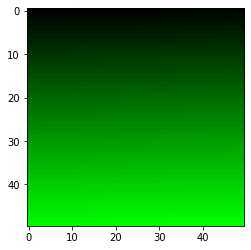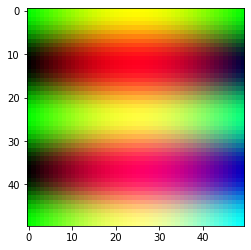# Mahotas – Creating RGB Image

• Last Updated : 10 Jul, 2020

In this article we will see how we can create a RGB image in mahotas. An RGB image, sometimes referred to as a truecolor image, is stored in MATLAB as an m-by-n-by-3 data array that defines red, green, and blue color components for each individual pixel. RGB image can be created with the help of array of each channel.

In order to do this we will use `as_rgb` method

Syntax : mahotas.as_rgb(r, g, b)

Argument : It takes three numpy array as argument

Return : It returns RGB ndarray object

Below is the implementation

 `# importing required libraries``import` `mahotas``import` `mahotas.demos``from` `pylab ``import` `gray, imshow, show``import` `numpy as np`` ` `# creating array of shape 50x50``# for red channel``r ``=` `np.arange(``2500``).reshape(``50``, ``50``)`` ` `# for blue channel``g ``=` `np.arange(``2500``).reshape(``50``, ``50``)`` ` `# for blue channel``b ``=` `np.arange(``2500``).reshape(``50``, ``50``)`` ` `# making red channel values to 0``r ``=` `r ``*` `0`` ` `# increasing green channel values``g ``=` `g ``*` `100`` ` `# making blue channel values to 0``b ``=` `b ``*` `0`` ` `# creating rgb image from these three channel``img ``=` `mahotas.as_rgb(r, g, b)`` ` `# showing image``imshow(img)``show()`

Output :Another example

 `# importing required libraries``import` `mahotas``import` `mahotas.demos``from` `pylab ``import` `gray, imshow, show``import` `numpy as np`` ` `# creating numpy linspace``z1 ``=` `np.linspace(``0``, np.pi)`` ` `# creating numpy meshgrid``X, Y ``=` `np.meshgrid(z1, z1)`` ` `# creating rgb channels``# creating red channel through sin function``red ``=` `np.sin(X)`` ` `# creating green channel through cos function``green ``=` `np.cos(``4` `*` `Y)`` ` `# creating blue channel``blue ``=` `X ``*` `Y`` ` `# creating rgb image from these three channel``img ``=` `mahotas.as_rgb(red, green, blue)`` ` `# showing image``imshow(img)``show()`

Output :My Personal Notes arrow_drop_up